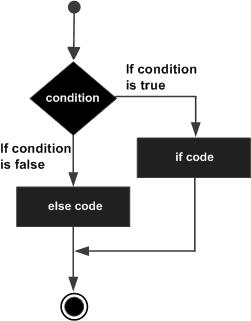# Powershell - If Else Statement

An if statement can be followed by an optional else statement, which executes when the Boolean expression is false.

## Syntax

Following is the syntax of an if...else statement −

```if(Boolean_expression) {
// Executes when the Boolean expression is true
}else {
// Executes when the Boolean expression is false
}
```

If the boolean expression evaluates to true, then the if block of code will be executed, otherwise else block of code will be executed.

## Flow Diagram## Example

```\$x = 30

if(\$x -le 20){
write-host("This is if statement")
}else {
write-host("This is else statement")
}
```

This will produce the following result −

## Output

```This is else statement
```

## The if...elseif...else Statement

An if statement can be followed by an optional else if...else statement, which is very useful to test various conditions using single if...elseif statement.

When using if, elseif, else statements there are a few points to keep in mind.

• An if can have zero or one else's and it must come after any elseif's.

• An if can have zero to many elseif's and they must come before the else.

• Once an else if succeeds, none of the remaining elseif's or else's will be tested.

## Syntax

Following is the syntax of an if...else statement −

```if(Boolean_expression 1) {
// Executes when the Boolean expression 1 is true
}elseif(Boolean_expression 2) {
// Executes when the Boolean expression 2 is true
}elseif(Boolean_expression 3) {
// Executes when the Boolean expression 3 is true
}else {
// Executes when the none of the above condition is true.
}
```

## Example

```\$x = 30

if(\$x -eq 10){
write-host("Value of X is 10")
} elseif(\$x -eq 20){
write-host("Value of X is 20")
} elseif(\$x -eq 30){
write-host("Value of X is 30")
} else {
write-host("This is else statement")
}
```

This will produce the following result −

## Output

```Value of X is 30
```
powershell_conditions.htm